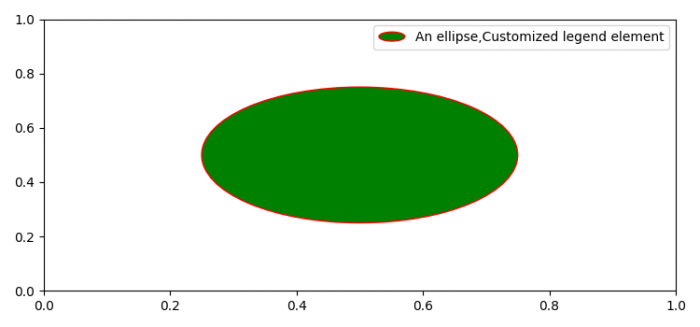# How to place customized legend symbols on a plot using Matplotlib?

To plot customized legend symbols on a plot, we can take the following steps −

• Set the figure size and adjust the padding between and around the subplots.
• Inherit HandlerPatch class, override create artists method, add an elliptical patch to the plot, and return the patch handler.
• Plot a circle on the plot using Circle class.
• Add a circle patch on the current axis.
• Use legend() method to place the legend on the plot.
• To display the figure, use show() method.

## Example

import matplotlib.pyplot as plt, matplotlib.patches as mpatches
from matplotlib.legend_handler import HandlerPatch

plt.rcParams["figure.figsize"] = [7.50, 3.50]
plt.rcParams["figure.autolayout"] = True

class HandlerEllipse(HandlerPatch):
def create_artists(self, legend, orig_handle, xdescent, ydescent, width, height, fontsize, trans):
center = 0.5 * width - 0.5 * xdescent, 0.5 * height - 0.5 * ydescent
p = mpatches.Ellipse(xy=center, width=width + xdescent, height=height + ydescent)
self.update_prop(p, orig_handle, legend)
p.set_transform(trans)
return [p]

c = mpatches.Circle((0.5, 0.5), 0.25, facecolor="green", edgecolor="red", linewidth=1)
plt.show()